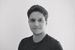Announcements
Product Release Webinar: Qlik Insider airing December 6! REGISTER TODAY!Employee

## Animate Charts using Nebula.js, Picasso.js, and D3.js Interpolation

Understanding d3-interpolate

The d3-interpolate module offers a way to compute intermediate values between two given values. The main interpolation method "d3.interpolate" takes two values as parameters (d3.interpolate(a, b)), and depending on the inferred type (numbers, colors, arrays, objects etc...), it routes them through the appropriate function that will create the relevant interpolator. This interpolator implementation is based on the type of the end value b.

For instance, if two strings representing CSS colors are passed to d3.interpolate, it will call the color interpolation method:

``````let color = d3.interpolate("blue", "red");
color(1) // will return #FF0000 the hex equivalent of red
color(0.5) // will return #800080, an purple-ish color that represents the mid color between blue and red``````

The same goes for interpolating between numbers, or even numbers inside strings. Take the following code for example where CSS transforms are interpolated, allowing us to make basic animations:

``````let transform = d3.interpolate("translate(0,0) rotate(0)", "translate(500, 50) rotate(90)");
transform(0.5) // returns translate(250, 25) rotate(45)``````

Animating a Picasso.js chart

Going back to our mashup, let's implement d3 interpolation within our nebula.js code, the goal is to animate a line chart in order to simulate a drawing effect of the line from left to right.

If you are not very familiar with how to build a chart using nebula.js and picasso.js, you can check out the official docs, this blog post, or this one which will walk you through all the building blocks to get up and running.

First things first, import the functions we need from their respective d3 modules, including the ease function, timer, and the interpolate function.

``````import { easeSinIn as ease } from 'd3-ease';
import { timer } from 'd3-timer';
import { interpolate } from 'd3-interpolate';``````

We then declare our transition variable and a way to reset it.

``````// Define transition & stopTransition function
let transition = null;

const stopTransition = () => {
if (transition) {
transition.stop();
transition = null;
}
};``````

Now for the fun part, we schedule a new timer to invoke the callback that includes our Picasso.js chart instance update function repeatedly until stopped.

The interpolate function takes an old and a new value and then a time argument from 0 to 1 and returns the right x and y coordinates which in our example results in the line animating.

``````      useEffect(() => {
if (!instance) {
return;
}

// reset transition
if (transition) {
stopTransition();
}

// Set duration
const duration = 5000;

// Run timer
transition = timer((elapsed) => {
// Set t
const t = Math.min(1, ease(elapsed / duration));

instance.update({
data: [
{
type: 'q',
key: 'qHyperCube',
data: layout.qHyperCube,
},
],
settings: {
scales: {
x: {
data: {
extract: {
field: 'qDimensionInfo/0',
},
},
},
y: {
data: { field: 'qMeasureInfo/0' },
invert: true,
expand: 0.1,
},
},
components: [
{
key: 'lines',
type: 'line',
data: {
extract: {
field: 'qDimensionInfo/0',
props: {
y: { field: 'qMeasureInfo/0' },
},
},
},
settings: {
coordinates: {
major: {
scale: 'x',
fn: (d) => {
const { items } = d.data;
const start = Math.max(0, d.datum.value - 1);
const end = d.datum.value;
const time = Math.min(1, (t - d.datum.value / items.length) * items.length);
return time < 0
? null
: interpolate(d.resources.scale('x')(start), d.resources.scale('x')(end))(time);
},
},
minor: {
scale: 'y',
fn: (d) => {
const { items } = d.data;
const start = items.find((item) => item.value === Math.max(0, d.datum.value - 1)).y.value;
const end = items.find((item) => item.value === d.datum.value).y.value;
const time = Math.min(1, (t - d.datum.value / items.length) * items.length);
return time < 0
? null
: interpolate(d.resources.scale('y')(start), d.resources.scale('y')(end))(time);
},
},
},
},
},
],
},
});

if (t === 1) {
stopTransition();
}
});
}, [layout, instance]);``````

That's all! Below is how the final result looks like. Keep in mind that this concept can be extended to be used with other Picasso.js components to achieve the animations you need, for instance interpolating the size of point components in bubble charts or box components in bar charts.

Please find the full code on Github for reference.Subscribe by Topic